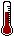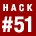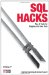# Hack 51. Display Rows As ColumnsStoring data in a normalized format often means having data in rows even when you must display it later in columns. This hack shows you how to turn that row-based data into columns.

Suppose you are responsible for students taking a program that includes many courses. It might be that you want to monitor their progress on a subset of these courses. Although many courses may be available, you are concerned with monitoring only the courses named Java, Database, and Algebra.

Something such as Table 7-6 would be useful for keeping track of your students' progress. It shows the data you want, column by column. However, the actual data is organized like Table 7-7, where the course results are shown row by row.

Table 7-6. Exam results output

Student Java Database Algebra
Gao Cong 80 77 50
Dongyan Zhou 62 95 62

Gao Cong Java 80
Gao Cong Database 77
Gao Cong Algebra 50
Dongyan Zhou Java 62
Dongyan Zhou Database 95
Dongyan Zhou Algebra 62

Let's look at two different ways to get the data into the required format.

7.3.1. Using a Self-Join

In this approach, you join the courseGrade table to itself three times. Each of these three instances of the table will select a different course. The java instance of the table shows only the Java results, the db instance has the Database values, and the alg instance has the Algebra grades. The join condition in each case ensures that each row of the output pulls a value from one of each of the three joined tables.

In the query shown, you can assume that there is a table or view called myStudents with a column called name:

```mysql> SELECT name, java.grade AS Java,
-> FROM myStudents
-> (name=java.student AND java.course='Java')
-> (name=db.student AND db.course='Database')
-> (name=alg.student AND alg.course='Algebra');
+--------------+------+------+---------+
| name | Java | DB | Algebra |
+--------------+------+------+---------+
| Gao Cong | 80 | 70 | 50 |
| Dongyan Zhou | 62 | 95 | 62 |
+--------------+------+------+---------+```

There is a potential weakness in this approach. If a student does not have a result for one of the four courses you will miss the entire row and the student will effectively be removed. You can support missing results and have a NULL value show in the appropriate cell.

By replacing each inner join with an outer join you can ensure that every student in the myStudents table will have a row in the result:

```mysql> DELETE FROM courseGrade WHERE student='Gao Cong' AND course='Algebra';
Query OK, 1 row affected (0.00 sec)

-> FROM myStudents
-> LEFT OUTER JOIN courseGrade java ON
-> (name=java.student AND java.course='Java')
-> LEFT OUTER JOIN courseGrade db ON
-> (name=db.student AND db.course='Database')
-> LEFT OUTER JOIN courseGrade alg ON
-> (name=alg.student AND alg.course='Algebra');
+--------------+------+------+---------+
| name | Java | DB | Algebra |
+--------------+------+------+---------+
| Dongyan Zhou | 62 | 95 | 62 |
| Gao Cong | 80 | 70 | NULL |
+--------------+------+------+---------+```

7.3.2. Using the CASE Statement

An alternative solution to this problem uses the CASE statement. The CASE statement allows you to perform IF/THEN/ELSE tests row by row. You set up a query that shows the course result or NULL for each row of the courseGrade table:

```mysql> SELECT name,
-> CASE WHEN course='Java'
-> THEN grade ELSE NULL END AS Java,
-> CASE WHEN course='Database'
-> THEN grade ELSE NULL END AS DB,
-> CASE WHEN course='Algebra'
-> THEN grade ELSE NULL END AS Algebra
-> FROM myStudents JOIN courseGrade ON (name=student);
+--------------+------+------+---------+
| name | Java | DB | Algebra |
+--------------+------+------+---------+
| Gao Cong | 80 | NULL | NULL |
| Gao Cong | NULL | 70 | NULL |
| Dongyan Zhou | 62 | NULL | NULL |
| Dongyan Zhou | NULL | 95 | NULL |
| Dongyan Zhou | NULL | NULL | 62 |
+--------------+------+------+---------+```

Notice there is one row for each row of the courseGrade table, each row has one valid result, and each column has one result per student. You can condense this table by grouping on the student name. The MAX function returns the highest number for each studentif all is well there will be, at most, one nonNULL value per student per column, and that number will MAX out all NULLs:

```mysql> SELECT name,
-> MAX(CASE WHEN course='Java'
-> THEN grade ELSE NULL END) AS Java,
-> MAX(CASE WHEN course='Database'
-> THEN grade ELSE NULL END) AS DB,
-> MAX(CASE WHEN course='Algebra'
-> THEN grade ELSE NULL END) AS Algebra
-> FROM myStudents JOIN courseGrade ON (name=student)
-> GROUP BY name;
+--------------+------+------+---------+
| name | Java | DB | Algebra |
+--------------+------+------+---------+
| Dongyan Zhou | 62 | 95 | 62 |
| Gao Cong | 80 | 70 | NULL |
+--------------+------+------+---------+```

If the student has not taken a course, a NULL value appears. If there is more than one result per student, each student will be given the highest mark.SQL Hacks
ISBN: 0596527993
EAN: 2147483647
Year: 2004
Pages: 147